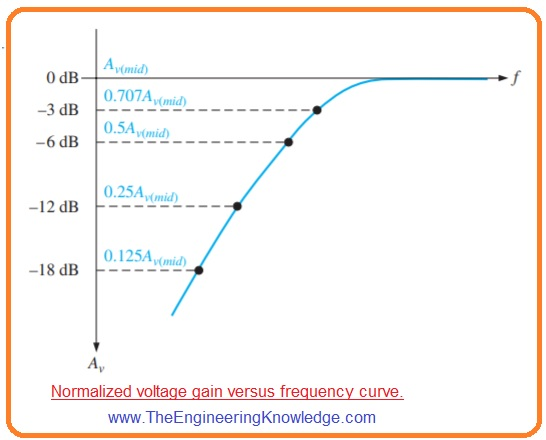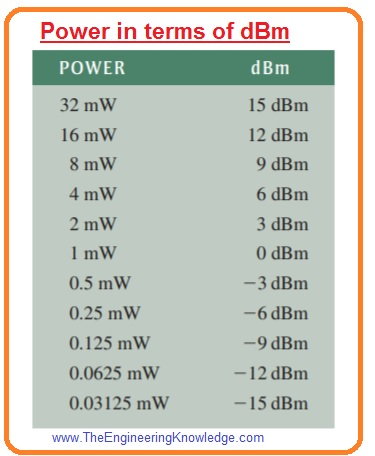Hello fellows, I hope you all are doing great. In today’s tutorial, we will have a look at How Decibel (dB) Express Amplifier Gain. A decibel is a unit of a logarithmic corresponding to one 10th of bel. It used to define the ratio between one value of power to other on a logarithmic scale. The logarithmic quantity is known as power level.

In today’s post, we will have a detailed look at how decibel expresses the gain of amplifier and its related parameters. So let’s get started with How Decibel (dB) Express Amplifier Gain.

#### How Decibel (dB) Express Amplifier Gain

• The decibel plays an important rule in measurements of amplifiers.
• The basics of the decibel unit originate from the logarithmic response of your ear to sound intensity.
• Decibel is logarithmic calculations of the ratio of one power to other or one voltage level to other.
• The power gain is defined in decibels with this given below formula.

Ap(dB)=10logAp

• Here Ap is real power gain which is Pout/Pin. Voltage gain is defined in decibels through this expression.

Av=(dB)20logAv

• If the value of Av is larger than one the dB gain has a positive value. If Av is lesser than one the dB gain is negative and known as attenuation.
• When you are working with these formulas use LOG key on your calculator.#### Zero dB Reference

• It is frequently appropriate in amplifiers to allocate a specific value of gain as zero dB reference.
• It does not mean that real voltage gain is one that is zero dB it mean that reference gain, does not matter what is actual value is used as a reference with which to relate other values of gain and is so allocated a zero dB value.
• Numerous amplifiers display a extreme gain over a specific range of frequencies and a decrease gain at frequencies less and greater this range.
• The highest gain exits for values of frequencies among higher and lower critical frequencies and is known as midrange gain that is define as zero dB.
• The value of gain less than midrange can be reference to zero dB and denoted as negative dB value.
• For instance if midrange voltage gain of specific amplifier is one hundred and the gain at specific frequency less than midrange is fifty then this decreased voltage can be explained as 20 log (50/100) = 20 log (0.5)= -6 dB
• It shows that it is six decibel less then zero dB reference.
• Splitting (halving) the output voltage for a steady input voltage is continually a six dB decrease in the gain.
• Similarly, a doubling of the output voltage is always a six decibel increment in gain.it shown in below figure.• This figure explained the normalized gain-vs-frequency curve displaying numerous decibels points.
• The word normalized means that the midrange voltage gain is allocated a value of one or zero decibels.
• The table shown in below figure shows doubling or halving voltage gains explains into the decibels values.
• you can note that when voltage gain is doubled decibel increases to six decibels and when gain is half decibels value reduces to six decibels.#### What is Critical Frequency

• The critical frequency also called cutoff frequency or corner frequency at which output power lost to the one half of its midrange value.
• It causes three decibel decrements in power gain as denoted in decibels through given expression.

Ap(dB) =10 log(0.5) = -3dB

• For critical frequency the value of voltage gain is 70.7 percent of its midrange value and denoted in decibels as.

Av(dB) =20 log (0.707) =-3dB

#### Power Calculation in dBm

• The dBm is unit used to calculate power level with reference to one milliwatt.
• Positive dBm quantities denotes power level over one milliwatt and negative dBm denotes power level less than one milliwatt.
• Since decibels can be used to denote only power ratios, not real power the dBm gives the easiest way to define real power output of amplifier or another instrument.
• Every three dBm increment relates to the doubling of power and three  dBm decrement relates to halving the power.
• To define that an amplifier has three decibel power gain denotes only that the output power is two time the input power and not related to the real output power.
• To denote actual output power the dBm can used.
• For instance three dBm is equal to the two milliwatt since two milliwatts is two time the one milliwatt reference.
• Six dBm is equal to the four milliwatt and it is continuous.
• Similarly -3dBm is like the 0.5 milliwatt. The below table indicates numerous values of power in the form of dBm.It is detailed post about How Decibel (dB) Express Amplifier Gain if you have any query ask in comments. Thanks for reading. Have a good day.